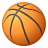# QlikView App Dev

Discussion Board for collaboration related to QlikView App Development.

Announcements
QlikWorld 2023, a live, in-person thrill ride. April 17 - 20, 2023, in Las Vegas! REGISTER TODAY
cancel
Showing results for
Did you mean:Not applicable

## Today Vs. Today-1 (Weekdays only) Balances

Hi,

I've got the below formula showing the difference Today Vs Yesterday.

However, when choosing a monday (for instance) the formula will compare balances Monday Vs. Sunday (no data, same as friday), so basically it will compare a balance monday with a zero balance sunday.

({\$<NumDate={\$(=max(NumDate))}>}Balance)-sum({\$<NumDate={\$(=max(NumDate)-1)},Date=>}Balance)

Num date is configured as below:

Num([date dd.mm.yyyy]) As NumDate

Can I use the (TempDate) as WeekDay  from my master calendar some way?

Olle

1 Solution

Accepted SolutionsMVP

Today

=SUM({<Date = {"\$(=Date(Max(Date),'DD.MM.YYYY'))"}>}Balance)

Yesterday

SUM({<Date = {"\$(=IF(WeekDay(Date(Max(Date),'DD.MM.YYYY'))='Mon',Date(Max(Date)-3,'DD.MM.YYYY'),

IF(WeekDay(Date(Max(Date),'DD.MM.YYYY'))='Sun',Date(Max(Date)-2,'DD.MM.YYYY'),

Date(Max(Date)-1,'DD.MM.YYYY'))))"}>}Balance)

Difference

=SUM({<Date = {"\$(=Date(Max(Date),'DD.MM.YYYY'))"}>}Balance) - SUM({<Date = {"\$(=IF(WeekDay(Date(Max(Date),'DD.MM.YYYY'))='Mon',Date(Max(Date)-3,'DD.MM.YYYY'),

IF(WeekDay(Date(Max(Date),'DD.MM.YYYY'))='Sun',Date(Max(Date)-2,'DD.MM.YYYY'),

Date(Max(Date)-1,'DD.MM.YYYY'))))"}>}Balance)

9 RepliesSpecialist

Try with NetworkdaysMVP

Use below without using NumDate

=SUM({<InvoiceDate = {"\$(=Date(Max(InvoiceDate),'DD.MM.YYYY'))"}>}Balance) - =SUM({<InvoiceDate = {"\$(=IF(WeekDay(Date(Max(InvoiceDate)-1,'DD.MM.YYYY'))='Sun',Date(Max(InvoiceDate)-2,'DD.MM.YYYY'),Date(Max(InvoiceDate)-1,'DD.MM.YYYY')))"}>}Balance)Not applicable
Author

Hi Manish. Returning Null values when I try it out.

Kind Regards,

OlleMVP

Provide sample data alongwith your requirements.Not applicable
Author

//OlleMVP

Today

=SUM({<Date = {"\$(=Date(Max(Date),'DD.MM.YYYY'))"}>}Balance)

Yesterday

SUM({<Date = {"\$(=IF(WeekDay(Date(Max(Date),'DD.MM.YYYY'))='Mon',Date(Max(Date)-3,'DD.MM.YYYY'),

IF(WeekDay(Date(Max(Date),'DD.MM.YYYY'))='Sun',Date(Max(Date)-2,'DD.MM.YYYY'),

Date(Max(Date)-1,'DD.MM.YYYY'))))"}>}Balance)

Difference

=SUM({<Date = {"\$(=Date(Max(Date),'DD.MM.YYYY'))"}>}Balance) - SUM({<Date = {"\$(=IF(WeekDay(Date(Max(Date),'DD.MM.YYYY'))='Mon',Date(Max(Date)-3,'DD.MM.YYYY'),

IF(WeekDay(Date(Max(Date),'DD.MM.YYYY'))='Sun',Date(Max(Date)-2,'DD.MM.YYYY'),

Date(Max(Date)-1,'DD.MM.YYYY'))))"}>}Balance)Not applicable
Author

Great stuff, thanks!Creator

Try to:

firstworkdate (max(NumDate), 1)

({\$<NumDate={\$(=max(NumDate))}>}Balance)-sum({\$<NumDate={\$(=firstworkdate (max(NumDate), 1) )},Date=>}Balance)

Ale.-Not applicable
Author

Hi Manish,

Now that I try to apply this to my master file, all of the three are returning zero-values. Script has the same structure as the sample file and the master calendar is identical. Any ideas of where to start looking?